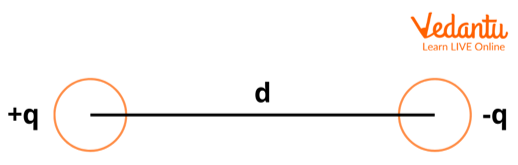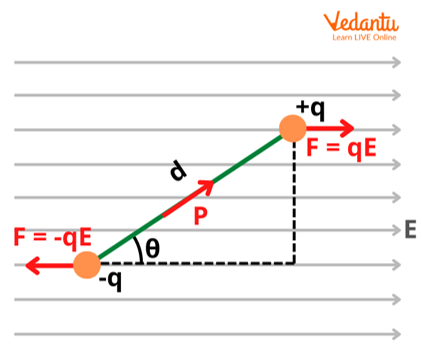Courses
Courses for Kids
Free study material
Free LIVE classes
More

# Potential Energy of an Electric Dipole for JEELIVE
Join Vedantu’s FREE Mastercalss

## What is an Electric Dipole?

An electric dipole moment is caused by two equal and opposite charges i.e., it consists of two charges of equal magnitude but opposite in direction. For instance, let’s consider two charges q and -q, which are separated from each other by a small distance d. The midpoint of the charges q and -q is the centre of the dipole and the charge in an electric dipole travels from negative to positive charge.  For a better understanding, look at the image given below.Electric Dipole Separated by Distance ‘d’

The combination of charge +q and -q in the above image can be stated as an electric dipole. Hence, in order for an electric dipole to exist, there must be two equal and opposite charges that are separated by a particular distance.

A few examples of electric dipoles include HCl, CH3COOH, H2O, etc. The centre of positive charge and negative charge do not coincide and as result, these molecules have a fixed dipole moment.

## Magnitude and Direction of an Electric Dipole Moment

The magnitude of an electric dipole moment is the product of the magnitude of one of the charges and the corresponding distance between them. It is denoted by $\overrightarrow{p}$. Mathematically, we write:

$\overrightarrow{p} = Q\overrightarrow{d}$

The direction of the dipole moment, in general, is from negative charge to positive charge. The axis of the dipole is the line along the direction of the electric dipole.

## Importance of Electric Dipoles

Molecules whose centre of positive charge and centre of negative charge coincide are called non-polar molecules as they lack polarity due to zero dipole moment. Methane and carbon dioxide are a few examples of non-polar molecules with zero dipole moment. On the other hand, molecules having a permanent dipole moment are called polar molecules.

The concept of an electric dipole is used in various fields like electrostatics, Physics, and Chemistry. For instance, it is useful in understanding phenomena such as surface tension, melting points, boiling points, solubility, and normal force.

Also, the dipole moment gives a measure of the tendency of various polar molecules to align in a certain direction in the presence of an external electric field. The weaker dipoles get out of alignment easily by external forces like vibration, temperature, etc.

## Electric Potential Energy of an Electric Dipole in a Uniform FieldElectric Dipole in an External Electric Field

Let’s consider a dipole consisting of two equal and opposite charges +q and -q, separated by a distance d in a uniform electric field of field intensity E. The force experienced by individual charges is +qE and -qE.

In the case of electric dipoles, instead of experiencing any force individually, they experience a torque when placed in a uniform electric field. The torque experienced by the electric dipoles is given by:

$\tau = P E$

Such a torque acts on the electric dipole only when the dipole is placed parallel or antiparallel to the external electric field.

Suppose an external torque which is equal in magnitude and opposite in direction is applied, it nullifies the effect caused by the torque due to the external electric field. As a result, the dipole is rotated without any angular acceleration at a minuscule angular speed. The work done is given by

Work done $W=PE(cos{{\theta }_{o}}-cos{{\theta }_{1}})$

By definition, the potential energy of any system is the work done in order to bring a system of charges from infinity to the desired position. Hence, in the case of electric dipoles in a uniform electric field, the potential energy is associated with the angle of inclination $\theta$ .

$U\left ( \theta \right ) = PE(cos\theta _{o} - cos\theta _{1})$

Since $cos\theta=0$ when the angle of inclination of the dipole with respect to the uniform electric field is 90 degrees, the potential energy at this angle for the electric dipole is also zero.

Considering the above case as the starting point i.e., starting with the angle of inclination at which potential energy is zero (90 degrees), the systems’ potential energy can be expressed as follows:

$U\left( \theta \right)=PE(cos\frac{\pi }{2}-cos\theta )$

$U =-PEcos\theta$

$U=-P.E$

Therefore, potential energy of an electric dipole in an uniform electric field will be equal to the product of the dipole moment and the electric field.

## Summary

An electric dipole consists of two charges with an equal magnitude but in opposite directions. It is important for these two charges not to coincide so that they form polar molecules which have various significant applications in the field of chemistry and physics. The potential energy of an electric dipole placed in a uniform external electric field is the amount of work done in bringing a system of charges from infinity to a desirable and related configuration.

Last updated date: 26th Sep 2023
Total views: 1.5k
Views today: 0.01k

## FAQs on Potential Energy of an Electric Dipole for JEE

1. When can dipole moments occur in molecules or ions? Comment.

Dipole moments can occur only if there is a separation between two charges. For instance, they can occur between atoms in a covalent bond or ions in an ionic bond. Dipole moments occur due to the difference in electronegativity of two atoms or ions. The dipole moment is a measure of the polarity and is decided by the distance between the two atoms or ions. This is different from the magnetic dipole moment where the object's spin is also an important deciding factor in calculating its magnitude.

2. How is electronegativity related to the electric dipole moment?

Dipole moment is a measure of the net polarity of molecules. Electronegativity is the tendency of atoms or molecules to attract electrons towards itself. The major factors that affect electronegativity are atomic number and the distance between valence electrons and the nuclei. If the electronegativities of bonded atoms are large, their dipole moments are also large. For example, NaCl is an ionic bond having the highest charge separation. Hence it has the highest dipole moment.